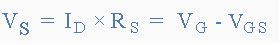## domingo, 21 de marzo de 2010

### JFET Amplifier

JFET Amplifier
So far we have looked at the Bipolar type amplifiers and especially the Common Emitter
amplifier, but small signal amplifiers can also be made using Field Effect Transistors or FET's. These devices have the advantage over bipolar devices of having an extremely high input impedance along with a low noise output making them very useful in amplifier circuits using very small signals. The design of an amplifier circuit based around a JFET (n-channel FET for this example) or even a MOSFET is exactly the same principle as that for a bipolar device and for a Class A amplifier as we looked at in the previous tutorial. A suitable Quiescent point still needs to be found for the correct biasing of the amplifier circuit with amplifier configurations of Common Source, Common Drain and Common Gate available for FET devices. In this tutorial we will look at the JFET Amplifier as a common source amplifier
as this is the most widely used design. Consider the Common Source JFET Amplifier circuit below.Common Source JFET Amplifier
The circuit consists of an N-channel JFET, but the device could also be an equivalent N-channel Depletion-mode MOSFET as the circuit diagram would be the same, just a change in the FET. The JFET Gate voltage Vg is biased through the potential divider network set up by resistors R1 and R2 and is biased to operate within its saturation region which is equivalent to the active region of the BJT. The Gate biasing voltage Vg is given as:Note that this equation only determines the ratio of the resistors R1 and R2, but in order to take advantage of the very high input impedance of the JFET as well as reducing the power dissipation within the circuit, we need to make these resistor values as high as possible, with values in the order of 1 to 10MΩ being common.
The input signal, (Vin) is applied between the Gate terminal and 0v with the Drain circuit containing the load resistor, Rd. The output voltage, Vout is developed across this load resistance. There is also an additional resistor, Rs included in the Source lead and the same Drain current also flows through this resistor. When the JFET is switched fully "ON" a voltage drop equal to Rs x Id is developed across this resistor raising the potential of the Source terminal above 0v or ground level. This voltage drop across Rs due to the Drain current provides the necessary reverse biasing condition across the Gate resistor, R2. In order to keep the Gate-source junction reverse biased, the Source voltage, Vs needs to be higher than the gate voltage, Vg. This Source voltage is therefore given as:Then the Drain current, Id is also equal to the Source current, Is as "No Current" enters the Gate terminal and this can be given as:This potential divider biasing circuit improves the stability of the common source JFET circuit when being fed from a single DC supply compared to that of a fixed voltage biasing circuit. Both Resistor, Rs and Capacitor, Cs serve basically the same function as the Emitter resistor and capacitor in the Common Emitter Bipolar Transistor amplifier circuit, namely to provide good stability and prevent a reduction in the signal gain. However, the price paid for a stabilized quiescent Gate voltage is that more of the supply voltage is dropped across Rs.

The basic circuit and characteristics of a common source JFET amplifier are very similar to that of the Common Emitter amplifier. A DC load line is constructed by joining the two points relating to the Drain current, Id and the supply voltage, Vdd intersecting the curves at the Q-point as follows.

JFET Amplifier Characteristics CurvesAs with the Common Emitter circuit, the DC load line produces a straight line equation whose gradient is given as: -1/(Rd + Rs) and that it crosses the vertical Id axis at a point equal to Vdd/(Rd + Rs). The other end of the load line crosses the horizontal axis at a point equal to Vdd. The actual position of the Q-point on the DC load line is determined by the mean value of Vg which is biased negatively as the JFET as a depletion-mode device. Like the bipolar common emitter amplifier the output of the Common Source JFET Amplifier is 1800 out of phase with the input signal.

One of the main disadvantages of using Depletion-mode JFET is that they need to be negatively biased. Should this bias fail for any reason the Gate-source voltage may rise and become positive causing an increase in Drain current resulting in failure of the Drain voltage, Vd. Also the high channel resistance, Rds(on) of the JFET, coupled with high quiescent steady state Drain current makes these devices run hot so additional heatsink is required. However, most of the problems associated with using JFET's can be greatly reduced by using enhancement-mode MOSFET devices instead.

Fuente: http://www.electronics-tutorials.ws/amplifier/amp_3.html
Asignatura: EES
Nombre: María José Nieto Cárdenas

#### 1 comentario:

1.you are clear my mind actually after reading your article i got clear my complete doubt. thanks for such easy understanding post. Sharing on what is pinch off voltage for a jfet for future aspect at here http:// electrotopic.com/what-is-the-pinch-off-voltage-for-a-jfet/